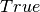Warning

This documents an unmaintained version of NetworkX. Please upgrade to a maintained version and see the current NetworkX documentation.

# from_pandas_dataframe¶

from_pandas_dataframe(df, source, target, edge_attr=None, create_using=None)[source]

Return a graph from Pandas DataFrame.

The Pandas DataFrame should contain at least two columns of node names and zero or more columns of node attributes. Each row will be processed as one edge instance.

Note: This function iterates over DataFrame.values, which is not guaranteed to retain the data type across columns in the row. This is only a problem if your row is entirely numeric and a mix of ints and floats. In that case, all values will be returned as floats. See the DataFrame.iterrows documentation for an example.

Parameters: df (Pandas DataFrame) – An edge list representation of a graph source (str or int) – A valid column name (string or iteger) for the source nodes (for the directed case). target (str or int) – A valid column name (string or iteger) for the target nodes (for the directed case). edge_attr (str or int, iterable, True) – A valid column name (str or integer) or list of column names that will be used to retrieve items from the row and add them to the graph as edge attributes. If, all of the remaining columns will be added. create_using (NetworkX graph) – Use specified graph for result. The default is Graph()

Examples

Simple integer weights on edges:

>>> import pandas as pd
>>> import numpy as np
>>> r = np.random.RandomState(seed=5)
>>> ints = r.random_integers(1, 10, size=(3,2))
>>> a = ['A', 'B', 'C']
>>> b = ['D', 'A', 'E']
>>> df = pd.DataFrame(ints, columns=['weight', 'cost'])
>>> df = a
>>> df['b'] = b
>>> df
weight  cost  0  b
0       4     7  A  D
1       7     1  B  A
2      10     9  C  E
>>> G=nx.from_pandas_dataframe(df, 0, 'b', ['weight', 'cost'])
>>> G['E']['C']['weight']
10
>>> G['E']['C']['cost']
9